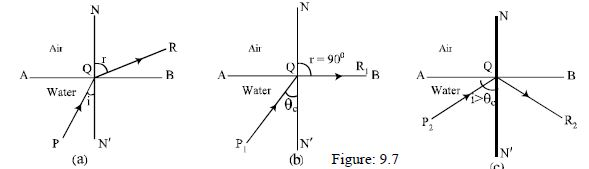# What is Critical Angle

When ray of light passes from a denser medium to a rarer medium, then the refracted my is deviated away from the normal. As a result the angle of refraction becomes greater than the angle of incidence.

Suppose AB is the separating surface of glass and air. Glass is denser and air is rarer medium. P is a point in the glass medium. From P a ray of light PQ incidents at point Q of separating surface AB at a small angle. QR is the refracted my in air [Fig: (a)]. In this case the angle of refraction (˄NQR) will be greater than the angle of incidence (˄PQN’).Now in denser medium if the angle of incidence gradually increases, the corresponding angle of refraction in rarer medium will also increase. In this way if the angle of incidence is increased finally for a definite angle of incidence ˄P1QN’ will be obtained [Fig: (b)] for which the refracted my QR will pass along the separating surface AB, i.e. the angle of refraction will be ˄NQR1 = 90°. In this situation the angle of incidence in the denser medium (˄P1QN’) is called the critical angle with respect to the rarer medium.

In fig: (b) ˄P1QN’ = θc = critical angle. The value of this critical angle also depends on the nature of the medium and the colour of light.